# 2D Shapes

Areas of Shapes

Circles

Circle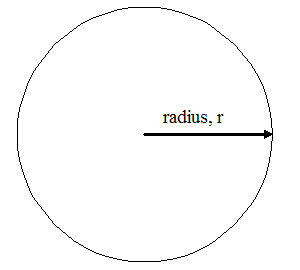Circumference of Circle: Formula: [ 2 * π * r ] or [ π* d ] Radius: units Result: units
 Area of Circle: Formula: [ π * r² ] or [ π * (d/2)² ] Radius: units Result: units²

Triangles

Triangle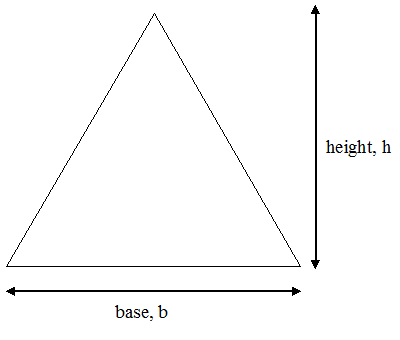Area of Triangle: Formula: [ 1/2 * b * h ] Length of base: units Height: units Result: units²

Square / Rectangle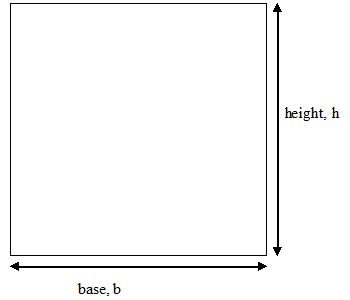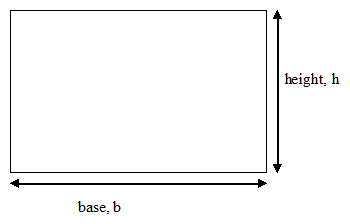Area of Square / Rectangle: Formula: [ b * h ] Length of base: units Height: units Result: units²

ParallelogramArea of Parallelogram: Formula: [ b * h ] Length of base: units Height: units Result: units²

Rhombus / Diamond / Kite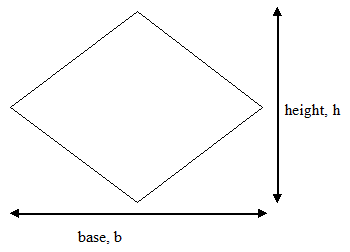Area of Rhombus / Diamond / Kite: Formula: [ 1/2 * b * h ] Length of base: units Height: units Result: units²

Trapezoid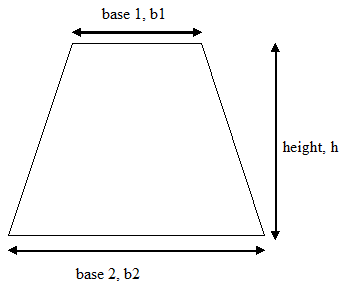Area of Trapezdoid: Formula: [ 1/2 * (b1 + b2) * h ] Length of base 1: units Length of base 2: units Height: units Result: units²